Intermediate Algebra

# 8.1Simplify Expressions with Roots

Intermediate Algebra8.1 Simplify Expressions with Roots

### Learning Objectives

By the end of this section, you will be able to:
• Simplify expressions with roots
• Estimate and approximate roots
• Simplify variable expressions with roots

### Be Prepared 8.1

Before you get started, take this readiness quiz.

1. Simplify: $(−9)2(−9)2$ $−92−92$ $(−9)3.(−9)3.$
If you missed this problem, review Example 2.21.
2. Round $3.8463.846$ to the nearest hundredth.
If you missed this problem, review Example 1.34.
3. Simplify: $x3·x3x3·x3$ $y2·y2·y2y2·y2·y2$ $z3·z3·z3·z3.z3·z3·z3·z3.$
If you missed this problem, review Example 5.12.

### Simplify Expressions with Roots

In Foundations, we briefly looked at square roots. Remember that when a real number n is multiplied by itself, we write $n2n2$ and read it ‘n squared’. This number is called the square of n, and n is called the square root. For example,

$132is read “13 squared”169 is called thesquareof 13, since132=16913 is asquare rootof 169132is read “13 squared”169 is called thesquareof 13, since132=16913 is asquare rootof 169$

### Square and Square Root of a number

Square

$Ifn2=m,thenmis thesquareofn.Ifn2=m,thenmis thesquareofn.$

Square Root

$Ifn2=m,thennis asquare rootofm.Ifn2=m,thennis asquare rootofm.$

Notice (−13)2 = 169 also, so −13 is also a square root of 169. Therefore, both 13 and −13 are square roots of 169.

So, every positive number has two square roots—one positive and one negative. What if we only wanted the positive square root of a positive number? We use a radical sign, and write, $m,m,$ which denotes the positive square root of m. The positive square root is also called the principal square root. This symbol, as well as other radicals to be introduced later, are grouping symbols.

We also use the radical sign for the square root of zero. Because $02=0,02=0,$ $0=0.0=0.$ Notice that zero has only one square root.

### Square Root Notation

$mis read “the square root ofm”.Ifn2=m,thenn=m,forn≥0.mis read “the square root ofm”.Ifn2=m,thenn=m,forn≥0.$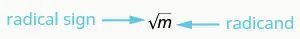We know that every positive number has two square roots and the radical sign indicates the positive one. We write $169=13.169=13.$ If we want to find the negative square root of a number, we place a negative in front of the radical sign. For example, $−169=−13.−169=−13.$

### Example 8.1

Simplify: $144144$ $−289.−289.$

### Try It 8.1

Simplify: $−64−64$ $225.225.$

### Try It 8.2

Simplify: $100100$ $−121.−121.$

Can we simplify $−49?−49?$ Is there a number whose square is $−49?−49?$

$()2=−49()2=−49$

Any positive number squared is positive. Any negative number squared is positive. There is no real number equal to $−49.−49.$ The square root of a negative number is not a real number.

### Example 8.2

Simplify: $−196−196$ $−64.−64.$

### Try It 8.3

Simplify: $−169−169$ $−81.−81.$

### Try It 8.4

Simplify: $−49−49$ $−121.−121.$

So far we have only talked about squares and square roots. Let’s now extend our work to include higher powers and higher roots.

Let’s review some vocabulary first.

$We write:We say:n2nsquaredn3ncubedn4nto the fourth powern5nto the fifth powerWe write:We say:n2nsquaredn3ncubedn4nto the fourth powern5nto the fifth power$

The terms ‘squared’ and ‘cubed’ come from the formulas for area of a square and volume of a cube.

It will be helpful to have a table of the powers of the integers from −5 to 5. See Figure 8.2.

Figure 8.2

Notice the signs in the table. All powers of positive numbers are positive, of course. But when we have a negative number, the even powers are positive and the odd powers are negative. We’ll copy the row with the powers of −2 to help you see this.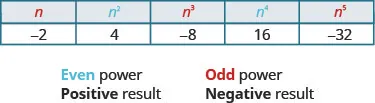We will now extend the square root definition to higher roots.

### nth Root of a Number

$Ifbn=a,thenbis annthroot ofa.The principalnthroot ofais writtenan.nis called theindexof the radical.Ifbn=a,thenbis annthroot ofa.The principalnthroot ofais writtenan.nis called theindexof the radical.$

Just like we use the word ‘cubed’ for b3, we use the term ‘cube root’ for $a3.a3.$

We can refer to Figure 8.2 to help find higher roots.

$43=6434=81(−2)5=−32643=4814=3−325=−243=6434=81(−2)5=−32643=4814=3−325=−2$

Could we have an even root of a negative number? We know that the square root of a negative number is not a real number. The same is true for any even root. Even roots of negative numbers are not real numbers. Odd roots of negative numbers are real numbers.

### Properties of $a n a n$

When n is an even number and

• $a≥0,a≥0,$ then $anan$ is a real number.
• $a<0,a<0,$ then $anan$ is not a real number.

When n is an odd number, $anan$ is a real number for all values of a.

We will apply these properties in the next two examples.

### Example 8.3

Simplify: $643643$ $814814$ $325.325.$

### Try It 8.5

Simplify: $273273$ $25642564$ $2435.2435.$

### Try It 8.6

Simplify: $1000310003$ $164164$ $2435.2435.$

In this example be alert for the negative signs as well as even and odd powers.

### Example 8.4

Simplify: $−1253−1253$ $164164$ $−2435.−2435.$

### Try It 8.7

Simplify: $−273−273$ $−2564−2564$ $−325.−325.$

### Try It 8.8

Simplify: $−2163−2163$ $−814−814$ $−10245.−10245.$

### Estimate and Approximate Roots

When we see a number with a radical sign, we often don’t think about its numerical value. While we probably know that the $4=2,4=2,$ what is the value of $2121$ or $503?503?$ In some situations a quick estimate is meaningful and in others it is convenient to have a decimal approximation.

To get a numerical estimate of a square root, we look for perfect square numbers closest to the radicand. To find an estimate of $11,11,$ we see 11 is between perfect square numbers 9 and 16, closer to 9. Its square root then will be between 3 and 4, but closer to 3.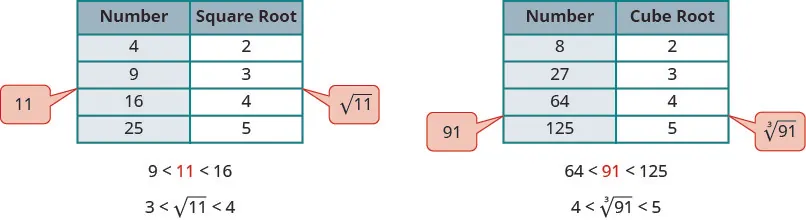Similarly, to estimate $913,913,$ we see 91 is between perfect cube numbers 64 and 125. The cube root then will be between 4 and 5.

### Example 8.5

Estimate each root between two consecutive whole numbers: $105105$ $433.433.$

### Try It 8.9

Estimate each root between two consecutive whole numbers:

$3838$ $933933$

### Try It 8.10

Estimate each root between two consecutive whole numbers:

$8484$ $15231523$

There are mathematical methods to approximate square roots, but nowadays most people use a calculator to find square roots. To find a square root you will use the $xx$ key on your calculator. To find a cube root, or any root with higher index, you will use the $xyxy$ key.

When you use these keys, you get an approximate value. It is an approximation, accurate to the number of digits shown on your calculator’s display. The symbol for an approximation is $≈≈$ and it is read ‘approximately’.

Suppose your calculator has a 10 digit display. You would see that

$5≈2.236067978rounded to two decimal places is5≈2.24934≈3.105422799rounded to two decimal places is934≈3.115≈2.236067978rounded to two decimal places is5≈2.24934≈3.105422799rounded to two decimal places is934≈3.11$

How do we know these values are approximations and not the exact values? Look at what happens when we square them:

$(2.236067978)2=5.000000002(2.24)2=5.0176(3.105422799)4=92.999999991(3.11)4=93.54951841(2.236067978)2=5.000000002(2.24)2=5.0176(3.105422799)4=92.999999991(3.11)4=93.54951841$

Their squares are close to 5, but are not exactly equal to 5. The fourth powers are close to 93, but not equal to 93.

### Example 8.6

Round to two decimal places: $1717$ $493493$ $514.514.$

### Try It 8.11

Round to two decimal places:

$1111$ $713713$ $1274.1274.$

### Try It 8.12

Round to two decimal places:

$1313$ $843843$ $984.984.$

### Simplify Variable Expressions with Roots

The odd root of a number can be either positive or negative. For example,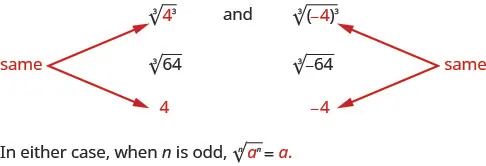But what about an even root? We want the principal root, so $6254=5.6254=5.$

But notice,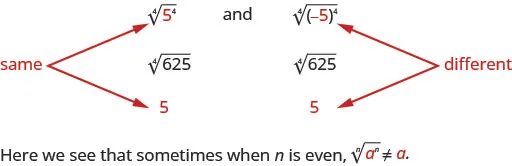How can we make sure the fourth root of −5 raised to the fourth power is 5? We can use the absolute value. $|−5|=5.|−5|=5.$ So we say that when n is even $ann=|a|.ann=|a|.$ This guarantees the principal root is positive.

### Simplifying Odd and Even Roots

For any integer $n≥2,n≥2,$

$when the indexnis oddann=awhen the indexnis evenann=|a|when the indexnis oddann=awhen the indexnis evenann=|a|$

We must use the absolute value signs when we take an even root of an expression with a variable in the radical.

### Example 8.7

Simplify: $x2x2$ $n33n33$ $p44p44$ $y55.y55.$

### Try It 8.13

Simplify: $b2b2$ $w33w33$ $m44m44$ $q55.q55.$

### Try It 8.14

Simplify: $y2y2$ $p33p33$ $z44z44$ $q55.q55.$

What about square roots of higher powers of variables? The Power Property of Exponents says $(am)n=am·n.(am)n=am·n.$ So if we square am, the exponent will become 2m.

$(am)2=a2m(am)2=a2m$

Looking now at the square root,

$a2mSince(am)2=a2m.(am)2Sincenis evenann=|a|.|am|Soa2m=|am|.a2mSince(am)2=a2m.(am)2Sincenis evenann=|a|.|am|Soa2m=|am|.$

We apply this concept in the next example.

### Example 8.8

Simplify: $x6x6$ $y16.y16.$

### Try It 8.15

Simplify: $y18y18$ $z12.z12.$

### Try It 8.16

Simplify: $m4m4$ $b10.b10.$

The next example uses the same idea for highter roots.

### Example 8.9

Simplify: $y183y183$ $z84.z84.$

### Try It 8.17

Simplify: $u124u124$ $v153.v153.$

### Try It 8.18

Simplify: $c205c205$ $d246d246$

In the next example, we now have a coefficient in front of the variable. The concept $a2m=|am|a2m=|am|$ works in much the same way.

$16r22=4|r11|because(4r11)2=16r22.16r22=4|r11|because(4r11)2=16r22.$

But notice $25u8=5u425u8=5u4$ and no absolute value sign is needed as u4 is always positive.

### Example 8.10

Simplify: $16n216n2$ $−81c2.−81c2.$

### Try It 8.19

Simplify: $64x264x2$ $−100p2.−100p2.$

### Try It 8.20

Simplify: $169y2169y2$ $−121y2.−121y2.$

This example just takes the idea farther as it has roots of higher index.

### Example 8.11

Simplify: $64p6364p63$ $16q124.16q124.$

### Try It 8.21

Simplify: $27x27327x273$ $81q284.81q284.$

### Try It 8.22

Simplify: $125q93125q93$ $243q255.243q255.$

The next examples have two variables.

### Example 8.12

Simplify: $36x2y236x2y2$ $121a6b8121a6b8$ $64p63q93.64p63q93.$

### Try It 8.23

Simplify: $100a2b2100a2b2$ $144p12q20144p12q20$ $8x30y1238x30y123$

### Try It 8.24

Simplify: $225m2n2225m2n2$ $169x10y14169x10y14$ $27w36z15327w36z153$

### Media

Access this online resource for additional instruction and practice with simplifying expressions with roots.

### Section 8.1 Exercises

#### Practice Makes Perfect

Simplify Expressions with Roots

In the following exercises, simplify.

1.

$6464$ $−81−81$

2.

$169169$ $−100−100$

3.

$196196$ $−1−1$

4.

$144144$ $−121−121$

5.

$4949$ $−0.01−0.01$

6.

$6412164121$ $−0.16−0.16$

7.

$−121−121$ $−289−289$

8.

$−400−400$ $−36−36$

9.

$−225−225$ $−9−9$

10.

$−49−49$ $−256−256$

11.

$21632163$ $25642564$

12.

$273273$ $164164$ $24352435$

13.

$51235123$ $814814$ $1515$

14.

$12531253$ $1296412964$ $1024510245$

15.

$−83−83$ $−814−814$ $−325−325$

16.

$−643−643$ $−164−164$ $−2435−2435$

17.

$−1253−1253$ $−12964−12964$ $−10245−10245$

18.

$−5123−5123$ $−814−814$ $−15−15$

Estimate and Approximate Roots

In the following exercises, estimate each root between two consecutive whole numbers.

19.

$7070$ $713713$

20.

$5555$ $11931193$

21.

$200200$ $13731373$

22.

$172172$ $20032003$

In the following exercises, approximate each root and round to two decimal places.

23.

$1919$ $893893$ $974974$

24.

$2121$ $933933$ $10141014$

25.

$5353$ $14731473$ $45244524$

26.

$4747$ $16331633$ $52745274$

Simplify Variable Expressions with Roots

In the following exercises, simplify using absolute values as necessary.

27.

$u55u55$ $v88v88$

28.

$a33a33$ $b99b99$

29.

$y44y44$ $m77m77$

30.

$k88k88$ $p66p66$

31.

$x6x6$ $y16y16$

32.

$a14a14$ $w24w24$

33.

$x24x24$ $y22y22$

34.

$a12a12$ $b26b26$

35.

$x93x93$ $y124y124$

36.

$a105a105$ $b273b273$

37.

$m84m84$ $n205n205$

38.

$r126r126$ $s303s303$

39.

$49x249x2$ $−81x18−81x18$

40.

$100y2100y2$ $−100m32−100m32$

41.

$121m20121m20$ $−64a2−64a2$

42.

$81x3681x36$ $−25x2−25x2$

43.

$16x8416x84$ $64y12664y126$

44.

$−8c93−8c93$ $125d153125d153$

45.

$216a63216a63$ $32b20532b205$

46.

$128r147128r147$ $81s24481s244$

47.

$144x2y2144x2y2$ $169w8y10169w8y10$ $8a51b638a51b63$

48.

$196a2b2196a2b2$ $81p24q681p24q6$ $27p45q9327p45q93$

49.

$121a2b2121a2b2$ $9c8d129c8d12$ $64x15y66364x15y663$

50.

$225x2y2z2225x2y2z2$ $36r6s2036r6s20$ $125y18z273125y18z273$

#### Writing Exercises

51.

Why is there no real number equal to $−64?−64?$

52.

What is the difference between $9292$ and $9?9?$

53.

Explain what is meant by the nth root of a number.

54.

Explain the difference of finding the nth root of a number when the index is even compared to when the index is odd.

#### Self Check

After completing the exercises, use this checklist to evaluate your mastery of the objectives of this section.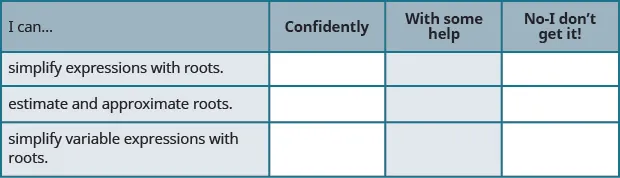If most of your checks were:

…confidently. Congratulations! You have achieved the objectives in this section. Reflect on the study skills you used so that you can continue to use them. What did you do to become confident of your ability to do these things? Be specific.

…with some help. This must be addressed quickly because topics you do not master become potholes in your road to success. In math every topic builds upon previous work. It is important to make sure you have a strong foundation before you move on. Who can you ask for help? Your fellow classmates and instructor are good resources. Is there a place on campus where math tutors are available? Can your study skills be improved?

…no - I don’t get it! This is a warning sign and you must not ignore it. You should get help right away or you will quickly be overwhelmed. See your instructor as soon as you can to discuss your situation. Together you can come up with a plan to get you the help you need.

Order a print copy

As an Amazon Associate we earn from qualifying purchases.